# THE Holographic Geometry Equations

## Introduction

At the 1982 International Symposium on Display Holography, two seminal papers on the Mathematics of Holography were presented, both by Steves, namely Benton and McGrew. This was the first time I had seen any work like this in understandable form, not like the crazed couple of pages in Principles of Holography by Howard M. Smith.

These two papers, plus a follow up in the succeeding 1985 ISDH proceedings by Benton, form the basis for computation of almost all the geometrical problems facing the display holographer. With these papers, the geometry of reference and object angles for pseudo-color holograms (multi-colored replay made with a single color laser) of the transmission and reflection configurations can be plotted. (I have also used them to successfully record HOE’s for a variety of applications.)

Alas and alack, these papers as originally written are only available in the out of print and hard to find Symposia proceedings. But Benton's papers have evolved into the digital age via his course notes, appropriately linked below, and McGrew posts his very interesting site at Graphical Methods

Benton’s approach is a trigonometric one, and McGrew’s is a geometric one; they are equivalent, giving the same result, except with Benton you plug and chug through equations, then map out the results. McGrew is more Euclidean; the diagram is made with straightedge and compass.

Benton is the master of the realm of the transmission hologram, and his equations are set up for reference and object beam coming from the same side. You can use them to find the alpha or tip angle of the master for registered multi-color rainbow holograms, along with where the RGB slits should be placed along it.

This may come as a surprise for those used to having the master hologram parallel to the copy plate when making reflection image plane holograms. But you can see from the McGrew diagrams that the light diffracted out of the H2 not only is dispersed, but the focus lies along a line that points back to the reference source! An H1 for a white light transmission hologram, either achromatic or rainbow, or a Benton hologram, (as He would prefer), is not aligned parallel to the subject!

There is another school of thought, where the master position is held constant, and the reference angle for the copy plate is varied, and you could get away with an H1 parallel to the copy. But then you have to kinematically reposition these H1s during recording, instead of all three slits on one plate, a much easier approach! In either case, these equations will solve that problem, too.

## The Equations

Let’s go through these equations with Adjunct Assistant Professor Ed Wesly as your guide. It would be a good idea to conjure up another instance of your browser to go to these sites and jump back and forth between the two.

The Benton equations are presented as part of one of his MIT courses, so let’s take a trip to Cambridge, Mass! (You may have to cut and paste these links into your second browser, as I am not so sure how successfully they will work.)

## Spatial Frequency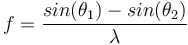Frequency, how often something happens, the frequencies most often coming to mind are the 20 to 20,000 cycles per second (abbreviated as Hertz) of good human hearing (not mine, for sure!) or the radio frequencies of 550 kiloHertz to 1800 kHZ of the AM band, or the GigaHertz processing speed of computer chips.

But Hertz, (named after Heinrich Hertz, the dude who proved the existence of radio waves after James Clerk Maxwell predicted them) involve changes or cycles per second, or units of time. For holographers, we are interested in units of space, hence spatial frequency.

Notice the units they are using: cycles per millimeter. Cycles because we are measuring complete phase changes, from a bright fringe to a dark fringe and back to the next bright fringe. Not individual fringes.

Millimeter because it is a convenient metric unit. If it’s not familiar to you, you’d better get on the stick.

What will cause some confusion in solving the upcoming equations is that because the units of spatial frequency are in cycles per mm, and our laser wavelengths are in nanometers, the latter have to be changed to the former. Milli- = thousandths, nano- = billionths, (micro- = millionths, inbetween) then there are a million nanometers in a millimeter. So the 633 nm of the He-Ne becomes .000633 mm in the equations, 515 nm Argon = .000515 mm, etc. Makes it much easier to use these decimal equivalents on the calculator than the scientific notation used in the examples.

Cycles per millimeter is roughly equivalent to the line pairs per millimeter figure of merit used in evaluating photographic objectives. (The difference is that the fringe cycle is a sinusoidal variation in intensity versus the high contrast of the test target.) A very decent normal lens as made in the heyday of 35 mm film photography by Nikon or Canon could resolve 100 line pairs per millimeter on a good day. This number would be found by photographing a standard test target, usually the 1951 USAF test target, which has a variety of black and white bars of varying sizes and spacings. Google it if unfamiliar with it.

Notice their sign convention: measured from the normal, with positive and negative angles. If you are not used to measuring from the normal, get with it! It is the standard of the optical industry. Not everything in optics is flat like a holo-plate; how can you measure an angle between a light ray and a convex lens or mirror surface? The normal is used, as you can generate a line that is at right angles to a circular surface at that point. Remember, there is that high school geometry saying, “a radius is at right angles to the circumference of a circle.”

I used to work with a pinhead who insisted on measuring the angles from the plate surface. Even though he had been one of my grad students at one time and should have known better, he had become perverted by working for a couple of schlockmeisters who did it the lazy way, and this bozo caused us some almost catastrophic failures by not following convention!

Another observation is that if you were making an unslanted grating (the fringes are at right angles to the holoplate, without a tilt) as they more or less show, with thetas 1 and 2 = 22.5 degrees, the fringe spacing would calculate differently than if you plugged in a reference theta of 45 and object theta of 0, even though there is an intra-beam angle of 45 degrees. What gives?

If you would make a moiré pattern using a pair of sheets of transparency material with parallel lines ruled on them (see the above image), and set a certain angle between them (45 degrees would make the spatial frequency too high to see) and counted the fringes per inch, you would get different counts depending on how you held the ruler. If the ruler were held perpendicular to the angle bisector of the sheets (Huh? Oh, like in the MIT illustration, the unslanted fringe case!) you would get a certain count. But if you held the ruler parallel to one of the rulings, you would get a lower count over the same distance from the same fringe system! The way this equation is set up takes into account the angle of the plate with respect to the incoming waves.

Related to this equation is finding the distance between fringes; instead of fringes per mm, how big is a fringe cycle? (= the distance from the center of one bright fringe to the next.) It is simply the inverse of the spatial frequency equation: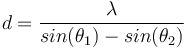(And don’t forget theta 2 is a negative angle, otherwise you will have an error!) (Or to make life easier, forget about the sign convention, and just add the sines of the two angles as positives together! But watch your step when getting too far off the track!)

Alternatively, to find the fringe spacing distance, you could simply use the 1/x button on the calculator once you have your spatial frequency. In either case the distance will be in millimeters, and the digits will usually all pop up behind the decimal point, so if you want to convert to microns, move the decimal point over three places to the right.

Onward and upward, as my Abstract Algebra teacher used to say. (while I nodded off!)

## Diffraction

We just went through Interference in the section above, next on this page is Diffraction. The way that this equation is written is asking the question “what is the output angle for a given grating with a particular input angle and wavelength?”

It is derived from the Interference equation by solving for the sine of one of the angles, but an extra term, m (an integer called order) pops up in this one. Let’s investigate how the two relate.

Looking at the MIT diagram for calculating spatial frequency,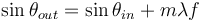They plug in the angles, and if you are doing this at home you end up with 1805 lines per mm, which they had rounded to 1800.

Substituting 40 degrees for theta in (a top lit holo in their diagram) and 1805 for f, lambda = 633 nm, same as we made it, that mysterious m set to 1 for right now, we get: (Notice that although I am considering this holo to be top referenced, this goofy MIT sign convention considers it a negative angle!)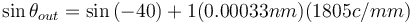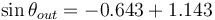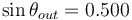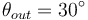Just like it should.

If we repeat the above but with m = 2, we get: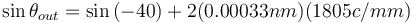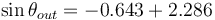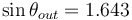Unh-unh! sin of an angle can’t be >1! (This is called a evanescent wave and is not propagated.)

So what is the role of m? Let’s make a grating with a lower spatial frequency. (an unslanted grating with beams at a small angle to the plate)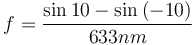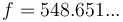(Damn, why can’t you cut and paste numbers from the Windows calculator into a document! And why does it disappear when I start typing!) Anyhow, let’s just round our spatial frequency to 550 cycles per mm.

Let’s do something different when interrogating this grating this time: after processing for ultimate efficiency, let’s hit the grating with a raw, undiverged He-Ne beam along the normal, so that way sin theta in disappears (sin 0 = 0) and our output equation becomes: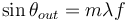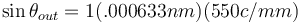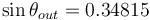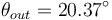Replacing m by 2,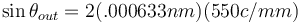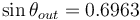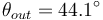When m = 3, we get a sine > 1, so we’re out of luck. But we could also replace m by -1 and -2, getting -20.4 and -44.1 degrees as more output angles.

This is the mathematics of low frequency gratings, kind of like those found in the diffraction grating goggles popular at fireworks displays etc., where you get multiple rainbows around sources of light.

To see where the other end of the rainbow comes out, let’s replace lambda by another popular Helium atmosphere laser, Helium-Cadmium, at 442 nm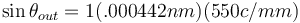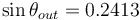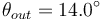Replacing m by 2,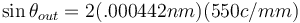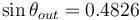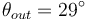This time we can get away by replacing m by 3,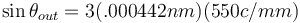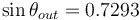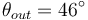So if we hit this grating with the He-Cd, dead nuts on at a right angle, we would see 7 beams: the straight through zero order, (see what happens when m = 0 in the above), two beams on either side of the normal at 14, 29 and 46 degrees (corresponding to m=-3,-2,-1,0,1,2 and 3)!

The red beams come out at 20 and 44 degrees. Need a picture? Get out the graph paper and draw it yourself!

If you have a diffraction grating, look at a light, and you will see, no matter what the spatial frequency, the blue or short wavelength end of the spectrum is closer to the light source than the red.

The best picture of the origin of the multiple orders is to be found in The Universe of Light, by Sir William Bragg. I have a 1963 Dover reprint which is pre-ISBN number, Google or Amazon.com it yourself. Pic is on p. 131 and 133, or click on this link to download the image, print it out, and follow the directions.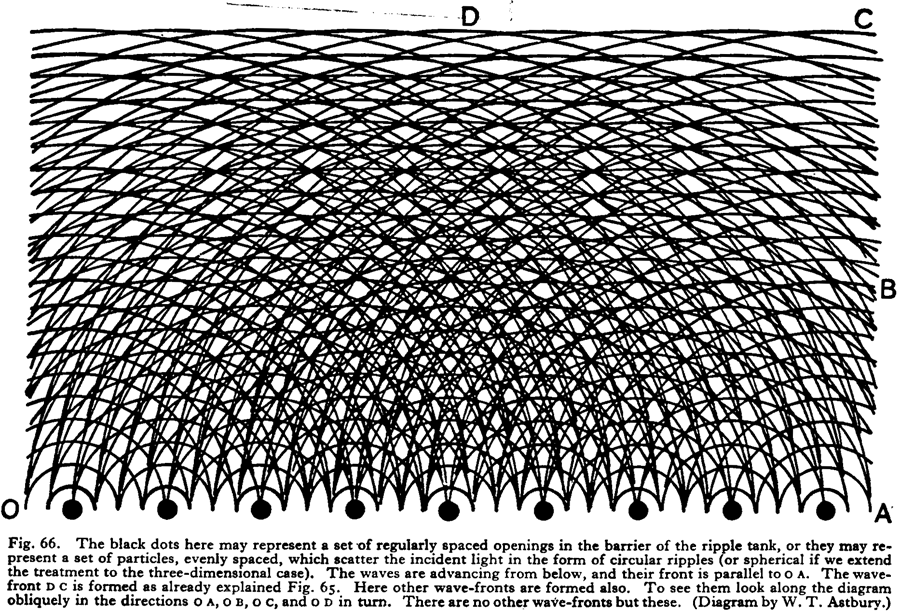## Angle Equation

This equation looks pretty imposing, but f in the above Diffraction Equation is replaced by the right hand side of the Interference Equation. It is used to describe the output angle of a point on an object when either the reference angle or the color of the reference beam is changed.

Instead of looking at an unslanted grating, for this equation, let’s look at a single point on a traditional transmission hologram. Reference angle is 45 degree from above, (-45 in this system) and the object point is right in the center of the hologram.

Preserving the recording parameters (putting the holo back in its plateholder and lighting it up under the exposure conditions):

Which is what we started with.

Moving the reference beam 5 degrees upward to 50 degrees:

We moved the reference beam upward 5 degrees, but the object only responded with a 3+ degree upward movement. This is the nature of relationships founded on sine curves.

Moving the reference beam 5 degrees downward to 40 degrees:

We moved the reference beam downward 5 degrees, and again the object only responded with a 3+ degree movement, this time down below the centerline we had established.

Little m, the order number, pops up in this equation again, but it is a rare contemporary hologram that exhibits higher order images. I have an early laser transmission hologram paper in my collection that boasts about a “Single Side Band Hologram” (sorry, need to find!) But even earlier, the original Gabor style holograms had lots of extra image orders or sidebands.

Some holograms in my collection are late ‘60’s/early 70’s Holex Holos. These exhibit sidebands. What happens is that when viewing these images, recorded with a shallow reference angle, there is the image that is immediately apparent in the center of the hologram, but moving off to either side of center there are higher order twin images that are visible, symmetric to the reference beam. This is analogous to the higher orders that were examined in the diffraction grating scenario above.

Let’s go back to those thrilling days of yesteryear when the modulation transfer function of recording materials (the ability to render higher spatial frequencies) may have been a concern and the reference angle was smaller, like 30 degrees from the normal and see what happens when m is increased.

Looking at an object point that is aligned along the normal, with a shallow ref angle:

Which is what we started with. All is well and good.

Searching for higher orders or sidebands:

This means that if this is a top lit holo, (ref angle negative in this nomenclature) we would not only see the image along the normal as expected, but another one when we stood on tippy-toes, coming up at 30 degrees from the normal.

Putting -1 and -2 into the above equation gives sines of angles = or > 1, so they don’t exist. But if the reference angle is less than 30, then there could be more images! But this means that you would most likely be looking at the reference beam while enjoying the first order object beam!

Let’s look at what happens when the illuminating or replay wavelength is different from the recording one. We will look at the hologram of the first example made with our He-Ne and reconstruct it with our frequency doubled YAG, 532 nm, going through the same optics.

Meaning the green image is above the red.

What the heck, let’s pretend we have a full-color set up. My favorite candidate for the blue primary is 476 nm of the Argon.

Meaning the blue image is above the green above the red, in other words closer to the reference source, as was noted in the diffraction grating examples.

When viewing a laser hologram with a white light, you can see a continuous spectral spread of objects, the blue part closer to the illuminating beam. If this hologram is illuminated with a mercury vapor street lamp, you can see several images displaced from each other, reconstructed by each of the major lines of the Mercury spectrum. Many antique laser transmission hologram displays used these kinds of light with a filter instead of (at that time more costly) laser.

An interesting lack of diffraction would occur when trying to illuminate H1’s for embossed rainbow holograms recorded at 458 nm with a 633 He-Ne. The geometry for this set up may be foreign for some, but it is standard operating procedure for registered color in the white light transmission mode. (The H1 plate was not parallel to the object, but tipped at the alpha or achromatic angle, and the collimated reference beam was at normal incidence to the plate!)

theta out = taboo! So we get no image!

## Horizontal Focus (1/R)

This is the most daunting of the equations we have seen so far; a fourth grader’s math nightmare; adding and subtracting fractions with unlike denominators. There are 4 R’s to be reckoned with; Radius of curvatures of reference wave, object point wave, illumination or replay light, and the output wave. Together with the previous Angle Equation, we can find the location of any object point when any of the recording or replaying parameters are transformed.

This equation is analogous to two lens equations, the first being the Lensmaker’s Equation, which takes into account the radius of curvature of both the front and back lens surfaces, their separation (or thickness of the lens) and the index of refraction of the material of the lens. In the holographic case, the (1/R obj - 1/R ref) term sets up what is known in Bentonese as the lambda focus. (It is not represented in this set of equations, but does pop up in his 1982 ISDH paper.)

The other lens equation incorporated into this holographic one is the Simple Lens Equation, which predicts the image position based on the object position and the intrinsic focal length of the lens.

1/focal length -1/object distance = 1/ image distance

Let’s run through an example with a collimated reference beam so that the 1/Rref drops out! (1/infinity = 0.) The object point will be placed 100 mm behind the holo plate, and we will start with a 633 nm He-Ne exposure. Since our reference angle will be 45 degrees, we won’t be seeing any second orders, so m will also disappear.

Like it should. This is what happens when we put the exposed holo back in the plateholder and illuminate it with the recording wavelength, 633 in this case.

Now let’s introduce the 532 nm beam into the set up like we did above:

Let’s just call it 119 mm.

So we are seeing the green image move back from the holo plate! We will couple that the results from the Angle Equation in a diagram below after doing the calculation for the blue.

Introducing the 476 nm beam into the set up like we did above:

Even further back! Combining these results with the angular displacements of the previous examples:

Putting all three into one picture:

A light gray is used for the reference path because the combined RGB beams would look white.

Another case where this equation is useful is in locating an object point’s position when the reference distance is changed. Using the hologram recorded above, 633 nm, 45 degree reference from infinity, and moving the reference in to 10 meters, the 100 mm object distance becomes:

If we get closer:

Even closer:

The object distance doesn’t change that dramatically when starting off with a collimated beam, but typical sandbox imagery has usually only about a meter or two for a reference beam path, let’s use a meter for ease of computation, 100 mm for object distance, and bringing the holo in to half a meter.

A quarter meter replay reference distance:

## Lateral Magnification

Lateral Magnification The ratio of the image distance to the object distance in lensed imagery gives the magnification ratio; how big is the image compared to its original size. There are a variety of equations useful for figuring this out, but the simplest to visualize and most like the holographic equation is:

When image distance = object distance, magnification ratio = 1:1, the Xerox machine case. In normal picture taking situations, a magnification < 1 or minification takes place; a whole human figure is reduced down to the size of a piece of film or digital chip, with object distance being much greater than image distance. (The denominator in the equation is bigger than the numerator.) A projector has a short object distance compared to its image distance, and the magnification is >1. (Values of numerator and denominator reversed.) Spare me and don’t ask about what happens when an object is at infinity today.

This equation refers to the object’s dimensions in the two planes parallel to the holoplate; the longitudinal, or z dimension magnification is an evil equation and is coming next. It relates the original object wavefront curvature, Robj to it’s current position, Rimg. But it can also apply to what happens when the replay wavelength is different than the exposing one. And our little friend m pops up and although not pertinent to the art of H1 masters it is useful for cases when there are multiple image orders, like in a Gabor hologram and its close relative, the zone plate.

So if we put our properly exposed and developed laser transmission hologram back in the plateholder where it was made once again like in the examples above, we can calculate:

Image is the same size as the object, since we haven’t moved anything.

In the previous section, the image distance was calculated when the reference beam was moved in closer. (Scenario refresher: reference beam in at 45 degrees from infinity or collimated, object plane 100 mm from plate.) Plugging in these object distances in response to moving in the reference beam closer, we get magnifications of these new object distances over 100 mm, since the ratio of wavelengths is unity and so is m.

• R ill = 10 m,
• R out = 99 mm;
• mag = .99
• R ill = 1 m,
• R out = 90.9 mm;
• mag = .909
• R ill = 100 mm,
• R out = 50 mm;
• mag = .5

If the object were a 10 by 10 grid of squares 10 mm (1 cm) on a side, the when the reference was at 10 m, the grids would shrink to 9.9 mm on a side; at 1 m, about 9.1 mm squares; and with that very close reference, they drop down to 5 mm mini-squares.

Couldn’t there be magnification just like in the lens examples above? There was none possible in the previous hologram because the reference beam during recording was collimated, parallel and coming from infinity, and there is no longer R ill to plug in and let the equation “grow” in replay.

Sharp-eyed readers might realize that there is no R ill in the Magnification Equation. But it’s necessary to find R img for the latter, and so the example will include solving for image distance based on reference replay position, then to be plugged into the magnification equation.

The parameters for this hologram will be Robj, the point on the object of interest, positioned 10 cm behind the holographic plate; and a Rref of one meter, a distance easily attainable in a sandbox or other small tabletop isolation system, for the reader to verify themselves. It could also be the basis for a Science Fair Project, something on the order of “Experimental Verification of Predicted Object Magnification in Transmission Holograms.” Or just looking at any transmission hologram by moving it back and forth in an expanding illuminating beam will also bring a sense of relevance to these mathematical meanderings.

1/R out = (lambda ill/lambda exp)(1/R obj - 1/R ref) + 1/R ill

= (633 nm/633 nm) X (1/100 mm - 1/1000 mm) + 1/1000 mm

= 1 X (.01 - .001) + .001

= .01,

R out = 100 mm

As usual this is the replay configuration that duplicates the recording, so the object distance in the hologram is the same as in real life, and it’s safe to say that magnification is = 1.

Halving the reference distance: 1/R out = (lambda ill/lambda exp)(1/R obj - 1/R ref) + 1/R ill

= (633 nm/633 nm) X (1/100 mm - 1/1000mm) + 1/500 mm

= 1 X (.01 - .001) + .002

= .011,

R out = 90 mm compared to R obj of 100 mm yields a magnification ratio of 90/100 = .9 or 90%. This didn’t yield an object magnification of 50%! This equation works exponentially, not linearly.

To see where the reference beam needs to come from for a magnification of 50%, (50 mm object distance, or R out) we need to solve for R ill: 1/R out = (lambda ill/lambda exp)(1/R obj - 1/R ref) + 1/R ill

= 1/50

= (633 nm/633 nm) X (1/100 mm - 1/1000 mm) +  ?

= .02

= 1 X .01 - .001 +  ?

1/R ill = .011,

R ill = 90.9 mm

Going in the other direction, doubling the R ill distance, 1/R out = (lambda ill/lambda exp)(1/R obj - 1/R ref) + 1/R ill

= (633 nm/633 nm) X (1/100 mm - 1/1000 mm) + 1/2000 mm

= 1 X (.01 - .001) + .0005

= .0095,

R out = 105.2631 mm, rounded to 105, for a 5% increase in image size.

The largest possible virtual image magnification would be when the R ill is brought all the way to infinity, so 1/R ill drops out, and the object distance is 1/.009, or 111.111…

An exercise for the reader would be to think of how to tweak the holographic set up (reference, object distance, and replay distance) for the maximum possible magnification, kind of like a holographic magnifying glass!

Another tricky transformation that this equation implies is the change in object size when the wavelength is changed. This was Gabor’s impetus for developing holography; to shoot the hologram with short wavelengths, like electron waves or X-rays, and then replay it in the optical domain for an extreme magnification, namely on the order of the ratio of the wavelengths. Replaying a hologram shot with 6 nm X-Rays with a 589 nm sodium vapor light (this is pre-laser era!) would yield approximately a 100X magnification!

Reading the early papers of Gabor and Rogers they did attempt to experimentally verify this by exposing holograms with the blue line of a mercury vapor lamp and replaying it with its green line! Theoretically satisfying, the big practical stumbling block was being able to supply a point source of the really short lambdas!

One of these eras I will finish this!

## Color Shifting of Reflection Holograms

The usefulness of the McGrew diagrams comes about when trying to make two different colored objects in the same image planed reflection hologram, using a chemical shifting technique with the plumping agent, TriEthanolAmine (TEA). Before attempting any of this more complicated multi-color business, the holographer should make a palette using various concentrations of TEA, as outlined in here.

If you do this, you would find that the unswelled sample, replaying in the original laser color, will reconstruct at the original reference angle. But you will also find that the shifted colors (I am assuming that the original color is in the red region, typically He-Ne 633 red, although this will work with any red. Starting in the green means you have to post-swell to get red, another, different, kind of headache!) as they go to shorter wavelength replay are not so bright at this same angle!

If you would lay your palette on the floor or table top, and position your replay light on the ceiling so that it lights up your unshrunk red most favorably, you would put the rest of them on the table above the red in ascending order of TEA concentrations, to get their brightest replay. The holograms are lit directly from page left in the photo below.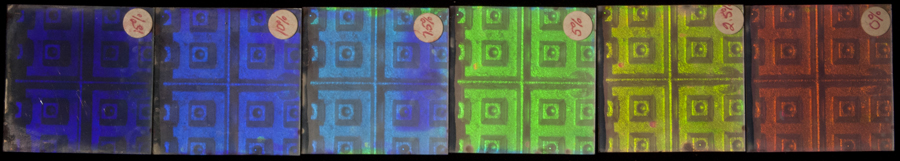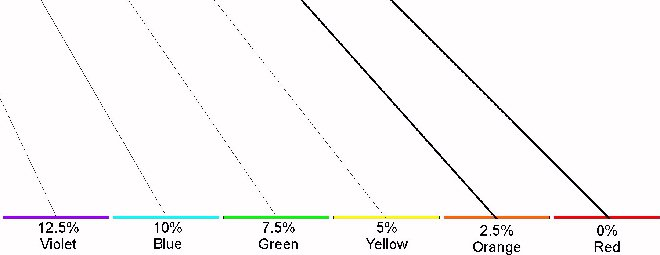This picture is not intended to be accurate, it is only to illustrate the trend: as the TEA concentration gets higher, with shrinkage to the bluer regions, the reference angle gets smaller. (Don't forget, the reference angle is measured from the normal, not the plate surface.)

The same fringe pattern is seen by the untreated control plate and the TEA-drenched plate to be shifted. If, after processing, the TEA treated one is resoaked in the same concentration, the fringes in the two holograms would be parallel. Without the expanding additive, the emulsion collapses down to its original out of the box thickness, not only compressing the spacing between the fringes to support a shorter wavelength, but changing their tilt. This tilt is in the direction of making the angle between the reconstructing (or illuminating) beam and the reconstructed beam smaller, as in the illustration above.

McGrew’s graphical reconstruction will allow the holographer to compensate for this change in replay conditions. In a nutshell, what needs to be done is to use larger reference angles during recording for the higher concentrations of TEA so that all images will replay with the same illumination angle after the shrinkage. Both Lon Moore and John Kaufman, pioneers of this technique, give their prop’s to Professor McGrew (although that term hadn’t come into usage in that pre-rapper era) in their papers in the same ISDH volume.

The only thing that these papers don’t predict is the loss of brightness with the change of angle. The holographer needs to tweak things by trial and error to balance colors properly. But the quasi-thick/thin behavior of the typical 6-7 micron emulsion of the commercial silver halide products is quite forgiving for most applications.